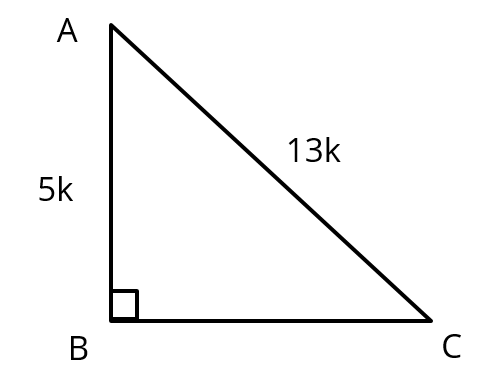# Extra Questions For Class 10 Maths Chapter 8 | Q4

###### Trigonometry | Trigonometric Values of Complementary AnglesClass 10 Math Questions

This CBSE class 10 Maths practice question is from the topic Trigonometry. It tests the concept of trigonometric values of complementary angles.

Question 4 : In ΔABC right angled at B, sin C = $$frac{\text{5}}{\text{13}}$. Find$i) sin A
(ii) cos A
(iii) cos C

## NCERT Solution to Class 10 Maths

### Explanatory Answer | Trigonometry Extra Question 4sin C = $$frac{\text{side opposite to ∠C}}{\text{hypotenuse}}$=$\frac{\text{AB}}{\text{AC}}$= $\frac{\text{5}}{\text{13}}$ ......$1)
Let AB = 5k and AC = 13k

#### Calculate Sine and Cosine value of the angles

Using Pythagoras theorem, BC = $$sqrt{AC^2− AB^2}$; BC = $\sqrt{$13k$^2− (5k)^2 }) = $$sqrt{169 k^2− 25 k^2 }$ = $\sqrt{144k^2 }$ = 12k sin A = $\frac{\text{side opposite to ∠A}}{\text{hypotenuse}}$= $\frac{\text{BC}}{\text{AC}}$= $\frac{\text{12k}}{\text{13k}}$= $\frac{\text{12}}{\text{13}}$ ......$2)
cos A = $$frac{\text{side adjcent to ∠ A}}{\text{hypotenuse}}$ = $\frac{\text{AB}}{\text{AC}}$= $\frac{\text{5k}}{\text{13k}}$= $\frac{\text{5}}{\text{13}}$ ......$3)
cos C = $$frac{\text{side adjcent to ∠ C}}{\text{hypotenuse}}$= $\frac{\text{BC}}{\text{AC}}$ = $\frac{\text{12k}}{\text{13k}}$ = $\frac{\text{12}}{\text{13}}$ ......$4)

Inference
From (1) and (3): sin A = cos C
From (2) and (4): sin C = cos A

#### Why?

If ABC is right angled at B, side adjacent to ∠ A will be the side opposite to ∠C.
Similarly side opposite to ∠A will be the side adjacent to ∠C.
i.e., ∠A = 90° - ∠C and ∠C = 90° - ∠A

###### Free CBSE Online CoachingClass 10 Maths

Register in 2 easy steps and
Start learning in 5 minutes!